# Appendix: Excel Solver Guide

Step 1: Click “file” in the header and open the “Options” menu: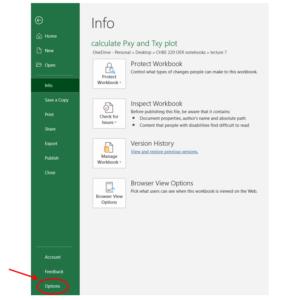Step 2: In the Excel Options menu, select “Solver Add-in” in the “Add-ins” section, then click “OK”.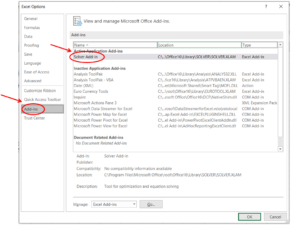Step 3: Then you can find the “Solver” in the “Analyze” section under the “Data” menu.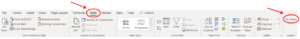Sample Question

A heat exchanger uses superheated steam to heat up a stream of pure acetic acid.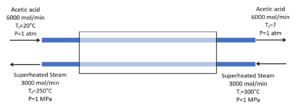The following data can be used for calculating the enthalpy change of the acetic acid stream:

$$C_{p}=A+BT+CT^2+DT^3$$

where T is in K and $C_{p}$ is given in J/mol K.

 A B C D acetic acid $4.84$ $0.2549$ $-1.753E-4$ $4.949E-8$

The enthalpy change for superheated steam can be found using data from the steam table:

 300°C, 1 MPa 250°C, 1 MPa $\hat{H}$ (J/mol) 54990 53035

The boiling point for acetic acid at 1 atm is 117.9°C. Does the acetic acid boil in the heat exchanger? If not, calculate the final temperature of the acetic acid stream. Assume there is no energy loss due to heat transfer.

## Solution

Because there are no other forms of energy transfer other than THE heat transfer between the streams, the energy balance simplifies to:

$$\Delta\dot{H}_{water}+\Delta\dot{H}_{acetic \;acid}=0$$

Based on the type of data given to calculate enthalpy change, the $\Delta\dot{H}$ term in the energy balance can be expanded to:

$$\Delta\dot{H}_{water}=\dot{H}_f-\dot{H}_i$$

\begin{align*}
\Delta\dot{H}_{acetic \;acid}&=\int_{T_i}^{T_f}C_pdT\\
&=\int_{T_i}^{T_f}A+BT+CT^2+DT^3 dT\\
&=AT+\frac{1}{2}BT^2+\frac{1}{3}CT^3+\frac{1}{4}DT^4\;\Bigg|_{T_i}^{T_f}\\
&= A(T_f-T_i)+\frac{1}{2}B(T_f-T_i)^2+\frac{1}{3}C(T_f-T_i)^3+\frac{1}{4}D(T_f-T_i)^4
\end{align*}

Step 1: Input given information that will be used in the calculation. This includes the given data to calculate enthalpy change, the molar flow rate of both substances, and the initial temperature of acetic acid.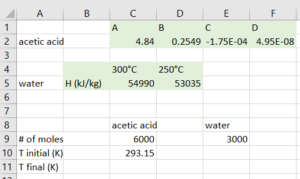Step 2: Calculate the molar enthalpy change. For water, this is calculated by subtracting the molar enthalpy values given at the final and initial temperatures. For acetic acid, we need to provide a guess of the final temperature to substitute into the equation.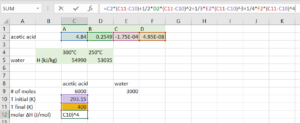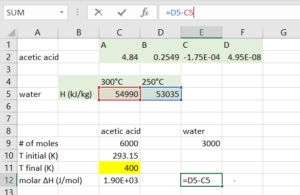Step 3: Calculate the total enthalpy change for both substances by multiplying the molar flow rate by the molar enthalpy change.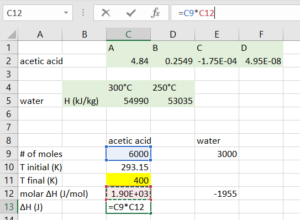ou

Step 4: Use a cell to represent the sum of enthalpy change. If we have guessed the correct final temperature, the value of this cell should be 0. We will use solver to let excel perform the guess-and-check process for us.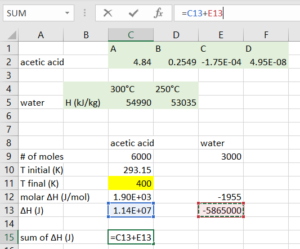Step 5: Select “Solver” from the “Data” menu in the header, and indicate that we want to solve for the final temperature of acetic acid that makes our $\text{sum of} \delta H=0$:

• Set objective to be the cell containing $\text{sum of} \delta H$
• To be: value of 0
• By changing variable cells containing the final temperature of acetic acid
• Click “Solve”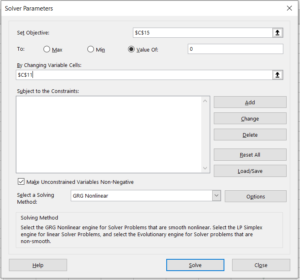Step 6: Click “OK” if solver finds a solution. If the solver cannot find a solution, it is maybe because our initial guess is too far away from the actual final temperature, if so, we can change the temperature and try to solve it again.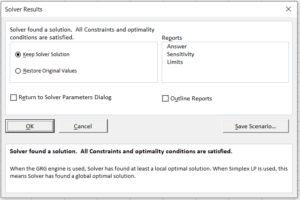Step 7: After the window closes, the value of the final temperature of acetic acid and all cells affected by temperature are changed to the corresponding values calculated with the actual final temperature.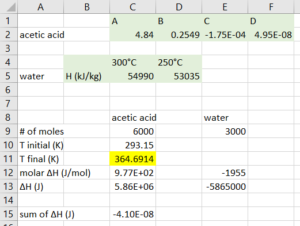Therefore, the final temperature of the acetic acid stream is $364.7K$, which is equal to $94.5°C$. This temperature is lower than the boiling temperature of acetic acid at 1 atm, so the stream will not start to boil.